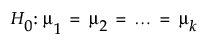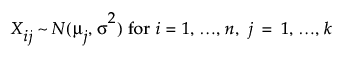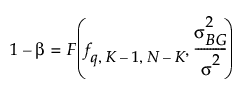Publication date: 11/10/2021

## Power for ANOVA

Use the Power Explorer for ANOVA to determine a sample size for a study of k groups or treatments to be analyzed using ANOVA. Select DOE > Sample Size Explorers > Power > Power for ANOVA. Explore the trade offs between variability assumptions, sample size, power and significance. Sample size and power are associated with the following hypothesis test:versus the two-sided alternative:

Ha : not all means equal

where:### Power Explorer for ANOVA Options

Set study assumptions and explore sample sizes using the radio buttons, text boxes, and sliders. The curve updates as you make changes to the settings. Alternatively, change settings by dragging the cross hairs on the curve or adjusting the values in the axis text boxes.

Fixed Parameters

Alpha

Specifies the probability of a type I error, which is the probability of rejecting the null hypothesis when it is true. It is commonly referred to as the significance level of the test. The default alpha level is 0.05.

Number of groups

Specifies the number of groups or treatments in your experiment.

Within-group variance (σ2)

Specifies the assumed population variance for each group where the standard deviation is assumed to be equal across groups.

Test Parameters

Parameters that are inter-related and update as you make changes.

Between-group variance

Specifies the variance of the individual group means around the grand mean.

Sample size (per group)

Specifies the number of observations (runs, experimental units, or treatments) needed for each group in your experiment.

Power

Specifies the probability of rejecting the null hypothesis when it is false. With all other parameters fixed, power increases as sample size increases.

Save Settings

Saves the current settings to the Saved Settings table. This enables you to save a set of alternative study plans. See Saved Settings in the Sample Size Explorers.

Make Data Collection Table

Creates a new data table that you can use for data collection.

### Statistical Details for the ANOVA Explorer

The power calculations for testing differences among means from multiple groups assuming equal standard deviations for each group is based on the standard F test. The power (1-β) is computed as follows:where

F(q, df1, df2, λ) is a non-central F-distribution with noncentrality parameter λ.

K is the number of groups

n is the number of samples within each group (assumed equal for all groups)

N = nK

σ2BG is the variation of the group means around the grand mean

σ2 is the within group variance (assumed equal for all groups)